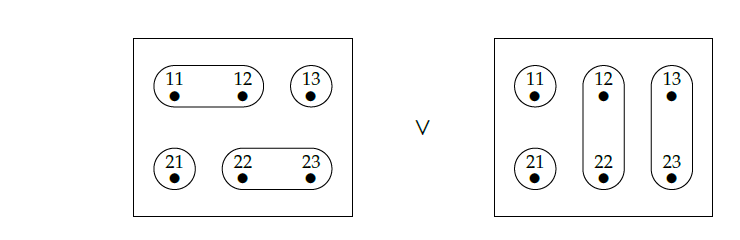> ) It would help if there would be a single page/cheat sheet of how to write formulas/charts and embed images into the posting (may be is there, but I just did not find it).) I tend to click on the gear-button to view other people's source and copy what I can,but that does not seem to work for many things.

I urge all you students to start creating such a cheat sheet. Add material to [[Forum - Guide]]! We've got 200 people here, including at least 100 computer scientists and programmers, so it should be quick work. I'll just give a few high-level hints.

**Writing math**

The Azimuth Forum uses [MathJax](https://docs.mathjax.org/en/latest/start.html#) to display equations. MathJaX is a version of LaTeX designed for use on the web, limited in its power compared to full LaTeX. If you don't know LaTeX, you can't do math in the 21st century! No other method is as good at creating equations such as this one from the book:

$$d(U,V) :=\sup_{u\in U}\inf_{v\in V}d(u,v) \qquad\qquad \mathcal{X}(U,V) :=\bigwedge_{u\in U}\bigvee_{v\in V}\mathcal{X}(u,v).$$

It takes a while to learn LaTeX: everyone serious about math must go through the pain of this. Here is a quick intro:

* [MathJax basic tutorial](https://math.meta.stackexchange.com/questions/5020/mathjax-basic-tutorial-and-quick-reference).

Unfortunately, a couple things on this tutorial don't apply here! Most importantly, as I mentioned on the welcome page:

For "displayed" equations, centered on the page, use double dollar signs: $$E = \sqrt{m^2 + p^2}$$ produces this: $$E = \sqrt{m^2 + p^2}$$

For "inline" equations, mixed in with your text, use this method: \$$E = \sqrt{m^2 + p^2}\$$ produces this: \$$E = \sqrt{m^2 + p^2}\$$.

**Formatting**

Unlike full-fledged LaTeX, MathJaX does not handle formatting _outside_ equations. The Azimuth Forum runs on [Vanilla](https://vanillaforums.com/en/features/forums/), but not all the fancy features of Vanilla have been activated here.

To create italics, boldface, quoted passages numbered or itemized lists, subject headers, and the like, use [Markdown](https://github.com/adam-p/markdown-here/wiki/Markdown-Cheatsheet). To include images you can also use HTML: for example,creates this:This image is stored in my own personal website. If someone wants to create a Github site or other method for storing images to be used in this course, please go ahead and let us know about it!

**Questions**

If you don't know how to do something, and someone else does, the quickest solution is often to click on the little gear at upper right of each comment, and choose "View Source". Then you can see how they did it.